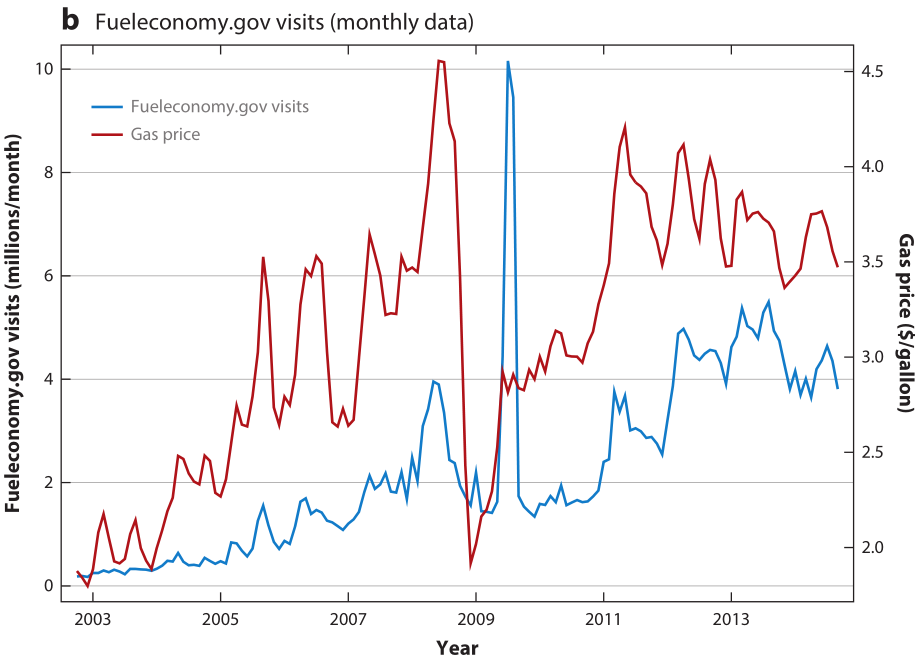## Energy Efficiency

[Econ 8852](http://www.richard-sweeney.com/ee_topics)
[Prof. Richard L. Sweeney](http://www.richard-sweeney.com/)

[to print slides, append "?print-pdf" to the url]

# Intro

Lecture draws on:

Allcott and Greenstone (JEP 2012) Allcott (Annual Review 2014) Gerarden, Newell and Stavins (JEL 2018)

### Energy services require capital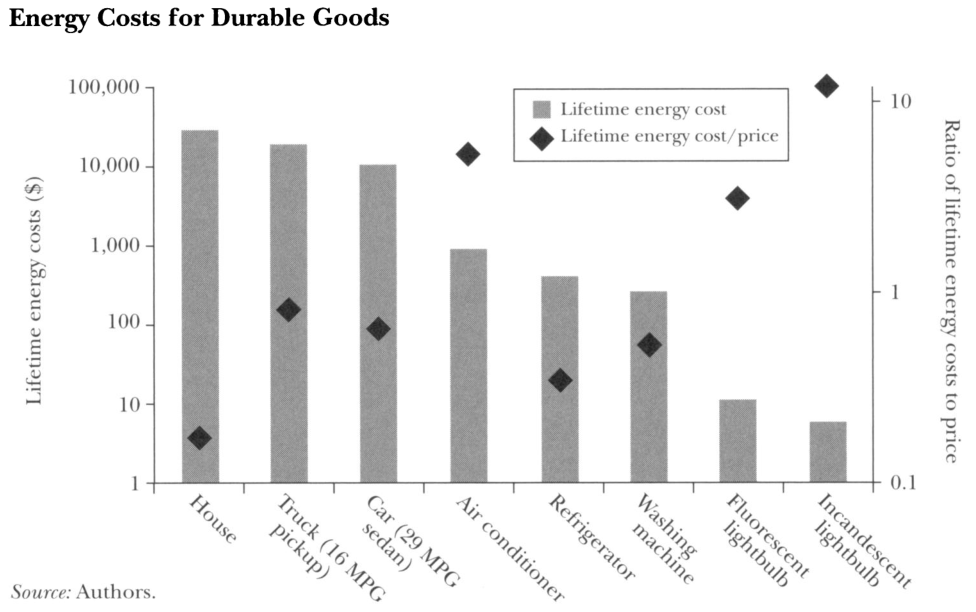### Long standing assertion that energy efficient capital suboptimally deployed

• Serious interest began during oil crisis in 1970s

• Engineers concluded that many energy saving technologies were slow to diffuse

Over time interest has centered on apparent “win-win” from environmental perspective

• Energy consumption associated with many externalities

### 40 years later this view still has a large audience### There are many “rational” reasons for EE gap

• agency issues
• ie landlord tenant
• resale issues
• home improvements
• engineering models wrong

### Research focussed on “behavioral” explanations

• [present bias]
• tradeoff is over cash flows not consumption
• car or mortgage payments also flows
• firm mispricing problem less clear
• car companies don’t sell you gasoline
• inattention/ salience
• Chetty et al (2009); Hossain \& Morgan (2006)
• cognitive issues /confusion
• Abaluck \& Gruber (2011); Allcott (2013)

### Setup

Consumers have indirect utility $u_{j} = \eta (y - p_j - \gamma g_j) + \nu_j$

• $p_j$ is upfront cost
• $\nu_j$ is the usage utility
• $g_j$ is the lifetime energy cost

Assuming $g$ is calculated and discounted appropriately, a natural test is to estimate $\gamma$ and test if its equal to 1.

$g_{j} = \sum\limits_{t=0}^{T} \delta^t m(r_j,e_t)r_j e_t$
• $m$ is utilization
• $r_j$ is energy requirement for capital $j$
• $e$ is the energy price

Note

• $\delta, m, T, e$ could all have $i$ subscripts
• if $m$ is endogenous, can lead to a “rebound effect”

### Early literature tried to estimate $\delta_i$

Hausman (1979) estimates a discrete choice model for AC’s

$u_{ij} = \eta (y_i - p_j - \delta_i \bar m_i r_j e_t) + \alpha X_j + \epsilon_{ij}$
• Has a small survey of households with submetered ACs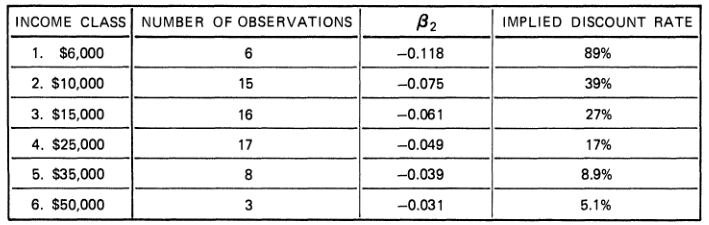### Literature then turned to within product variation

• In the cross section, concern that $E[r_j \epsilon_j] \ne 0$

However, $g_{ij}$ varies for many other reasons within product

• energy prices vary
• across regions, time
• lifetime varies at time of sale
• Sallee et al. look at mileage

## Allcott and Wozny

ReStat (2014)

• What’s the research question?
• What’s the empirical strategy?
• What data do they have?

### AW cost to own

$p_{j} + \sum\limits_{t=0}^{T} \delta^t m(r_j,e_t)r_j e_t$

• used car auction vehicle prices from Manhiem
• what are the assumptions using this?
• assumed real discount rate of 6%
• vehicle loan rate 6.9%
• S\&P avg return 5.9%
• (exogenous) VMT ($m$) and $T$ from NHTSA
• fuel economy from EPA
• national avg gasoline prices / oil futures

### Controlling for product quality clearly matters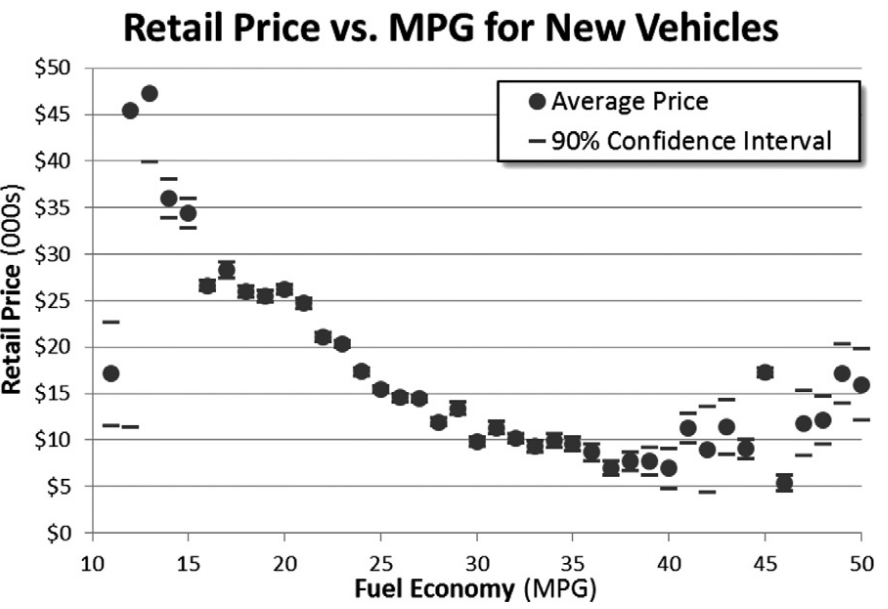### Identifying variation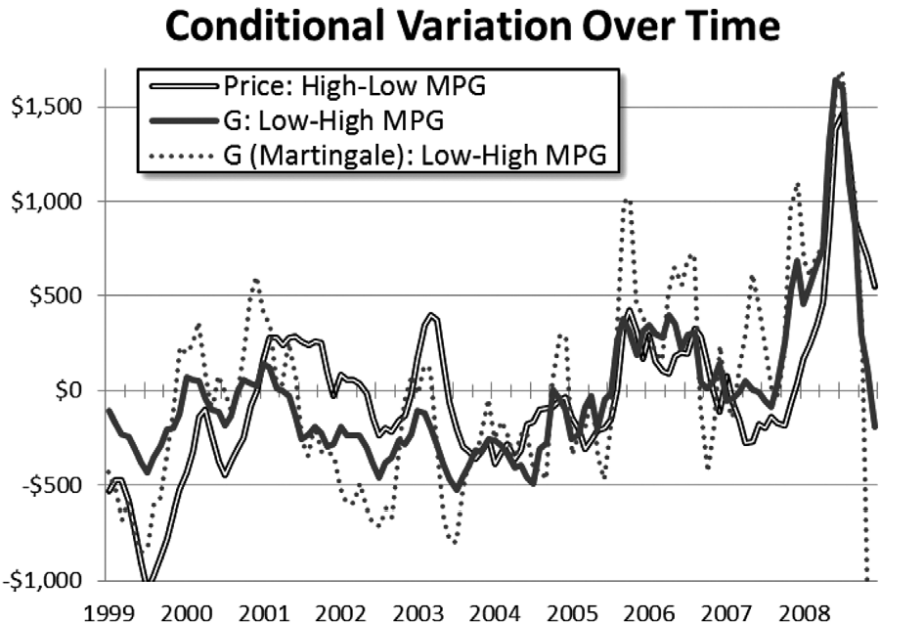### AW use hedonic regression

Typical logit share identity (Berry, 1994): $\ln s_{jat} - \ln s_{ot} = -\eta (p_{jat} - \gamma g_{jat}) + \psi_{ja} + \tilde \xi_{jat}$

AW actually estimate: $p_{jat} = - \gamma g_{jat} + \tau_t + \psi_{ja} + \epsilon_{jat}$

Why do they do this?

Berry equation rearranged is $p_{jat} = - \gamma g_{jat} - \frac{1}{\eta}(\ln s_{jat} - \ln s_{ot} ) + \psi_{ja} + \tilde \xi_{jat}$

• The don’t have shares.
• Absorb $s_{ot}$ with time FEs.
• Put product FEs in $\psi_{ja}$
• $\xi$ and the remaining share difference in the $\epsilon$

$p_{jat} = - \gamma g_{jat} + \tau_t + \psi_{ja} + \epsilon_{jat}$

• So this tests it tests whether relative vehicle prices move one-for-one with changes in the relative gasoline costs.
• Coeff of interest $\gamma$ recovered with OLS.

### AW also using a grouping estimator

• group all cars by month, above / below median

• $Z_{jat}^u = 1(f_{ja} > f^{50}) \times 1(t=u$)
• instrument for $G$ with $Z$

• why do they do this?

Jerry Hausman’s (2001) “iron law of econometrics”: due to measurement error, the magnitude of a parameter estimate is usually smaller in absolute value than expected

What is the null hypothesis that AW want to test?

$p_{jat} = - \gamma g_{jat} + \tau_t + \psi_{ja} + \epsilon_{jat}$

### Visual results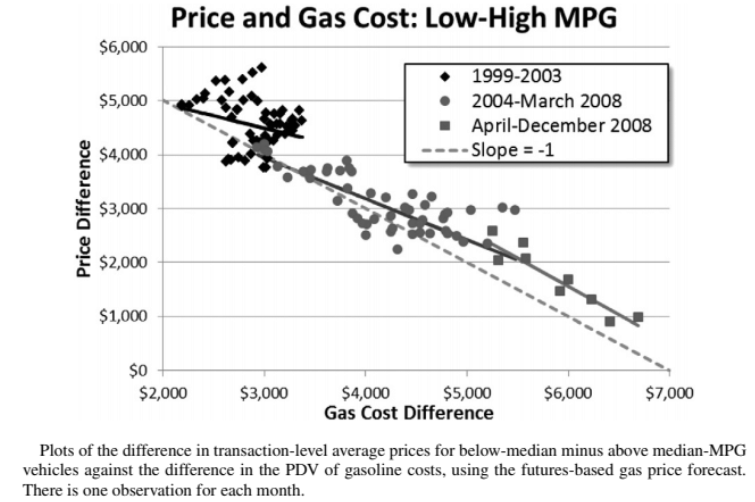### AW Results - Sensitivity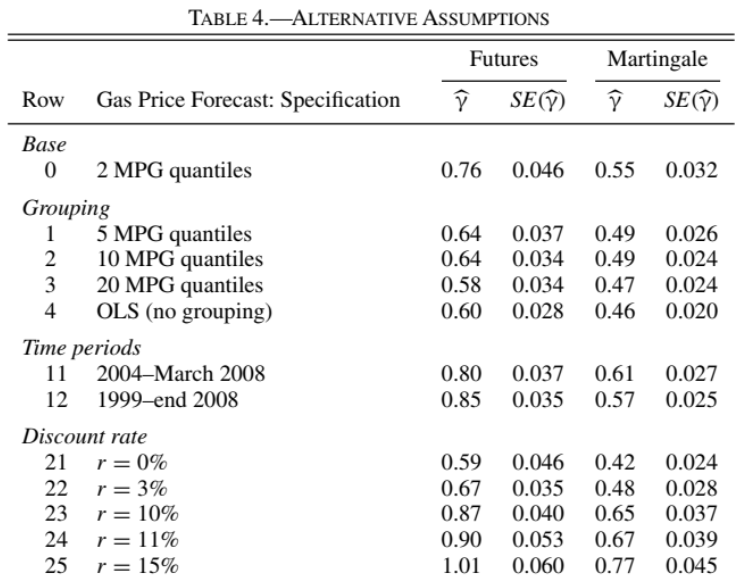### What do people takeaway from this paper?

• for new cars, close to 1
• old cars, very low
• results sensitive to how G is constructed

• are people making mistakes?

• what questions does this leave open?

## Allcott and Knittel

AEJ: Policy 2019

### Recent emphasis has been on experiments

• Many studies similar to AW

• Although panel data helps, interpretation still requires econometrician to construct $g$

• If hypothesis is that $\gamma < 1$ due to inattention, imperfect information or bounded rationality, an alternative is to experimentally vary exactly those margins

### Alternative: AK 2019 conduct two large field experiments

Experiment #1:

• Hang out at car dealers and intercept potential buyers
• Randomly explain fuel economy savings to some

Experiment #2:

• Conduct and online survey of people how say they are in the market for a new car
• In addition to following up on what people actually bought, AK elicit WTP for fuel economy.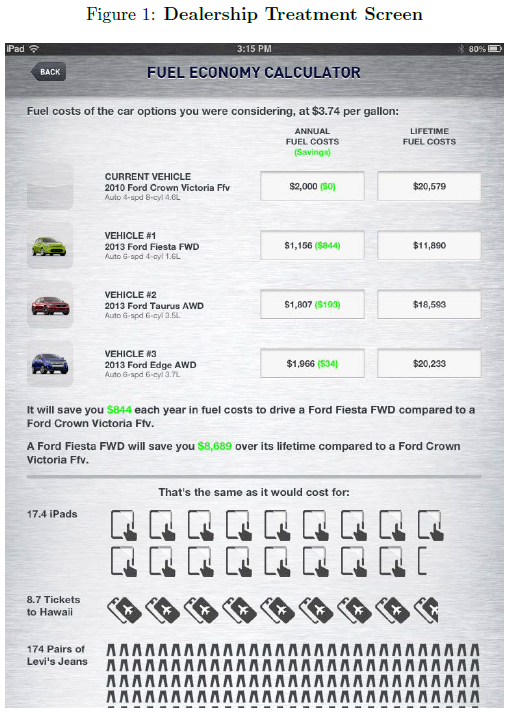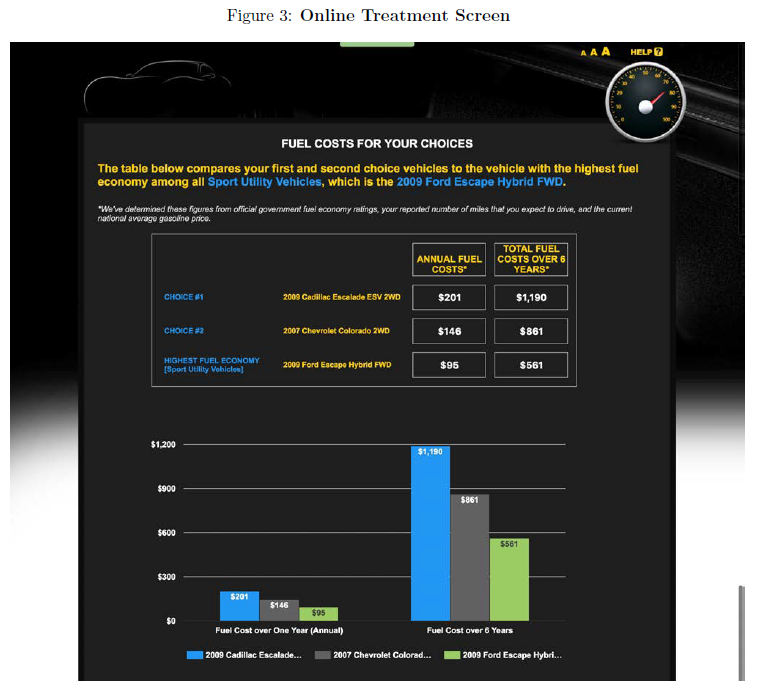• can elicit WTP

### Consideration sets reveal important differences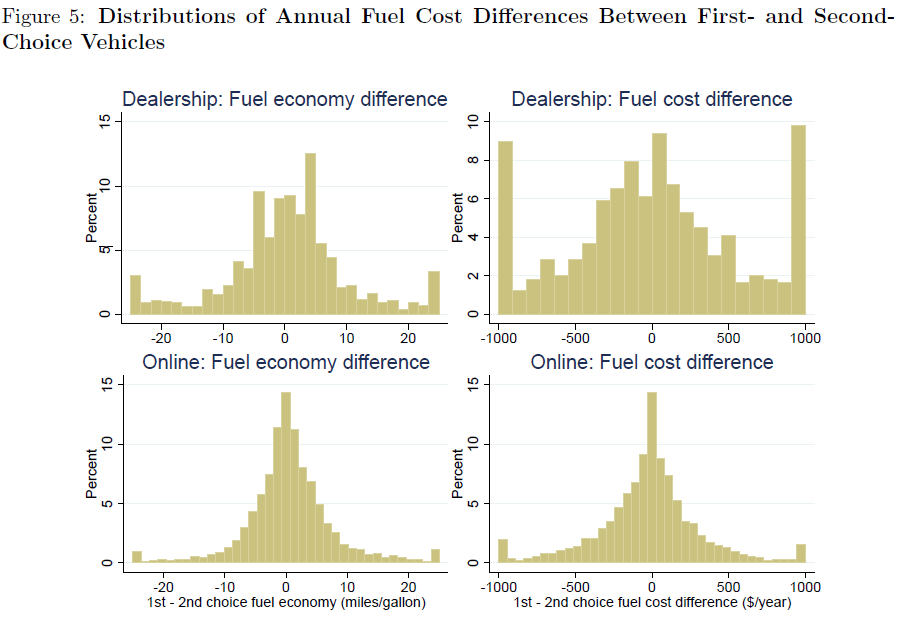### Beliefs about fuel costs noisy, but not biased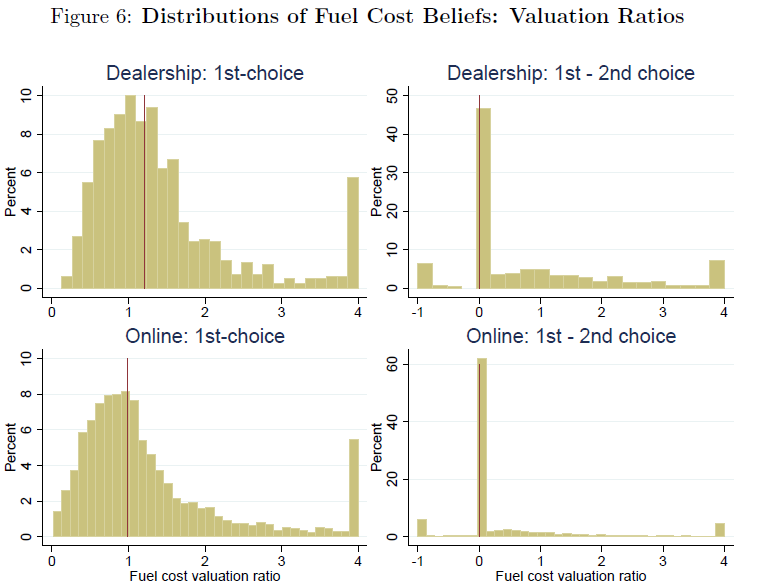### Results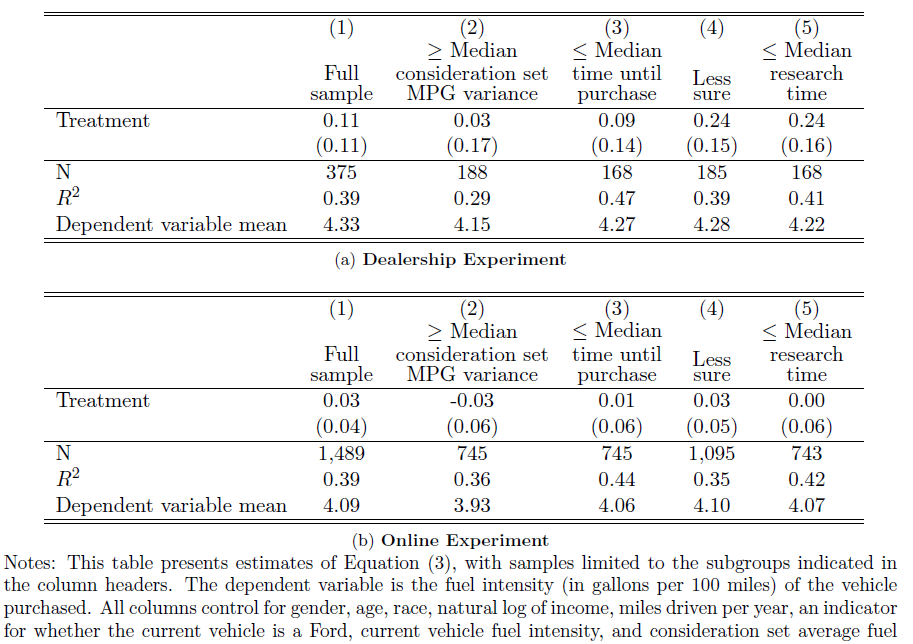## Policy Implications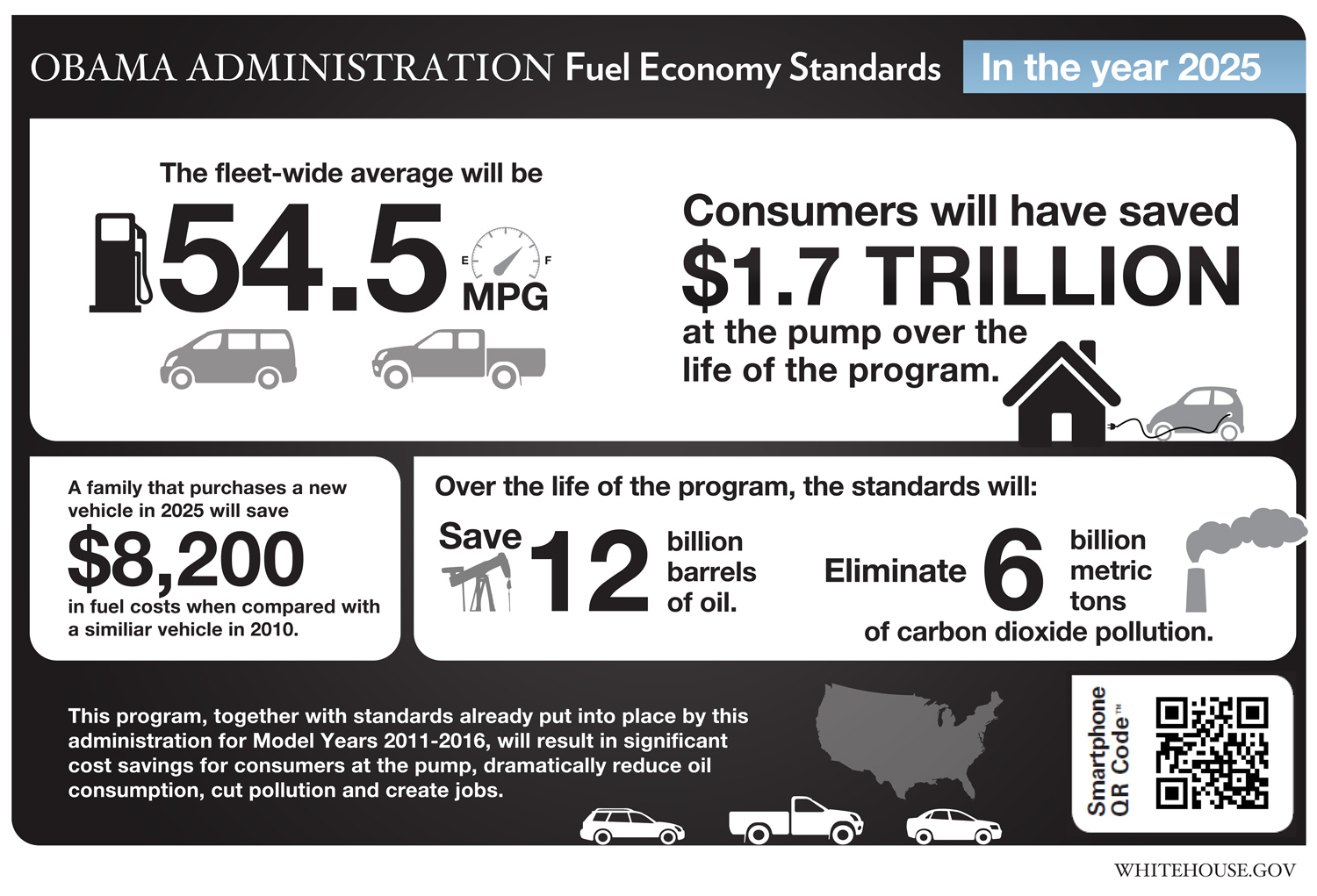### Some conclusions from core EE gap lit

• assertion that consumers making large mistakes on average appears incorrect

• once you account for product unobservables and use exogenous fuel cost variation, tradeoffs seem close to rational

• experimental studies directly educating or directing consumer attention to energy costs have found very small impacts

### Future directions

• Heterogeneity in both values and bias
• policy implications
• targeting
• What role do firms play here?
• Are they offering the “right” set of products?
• How do consumer’s form beliefs?
• we know the true calculation is hard

## Allcott and Taubinsky

AER 2015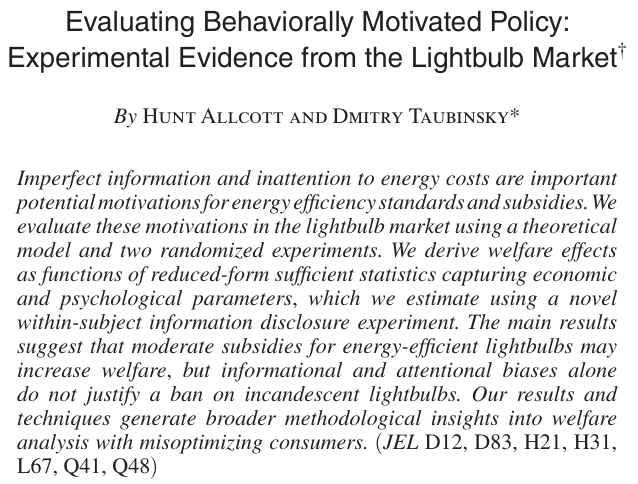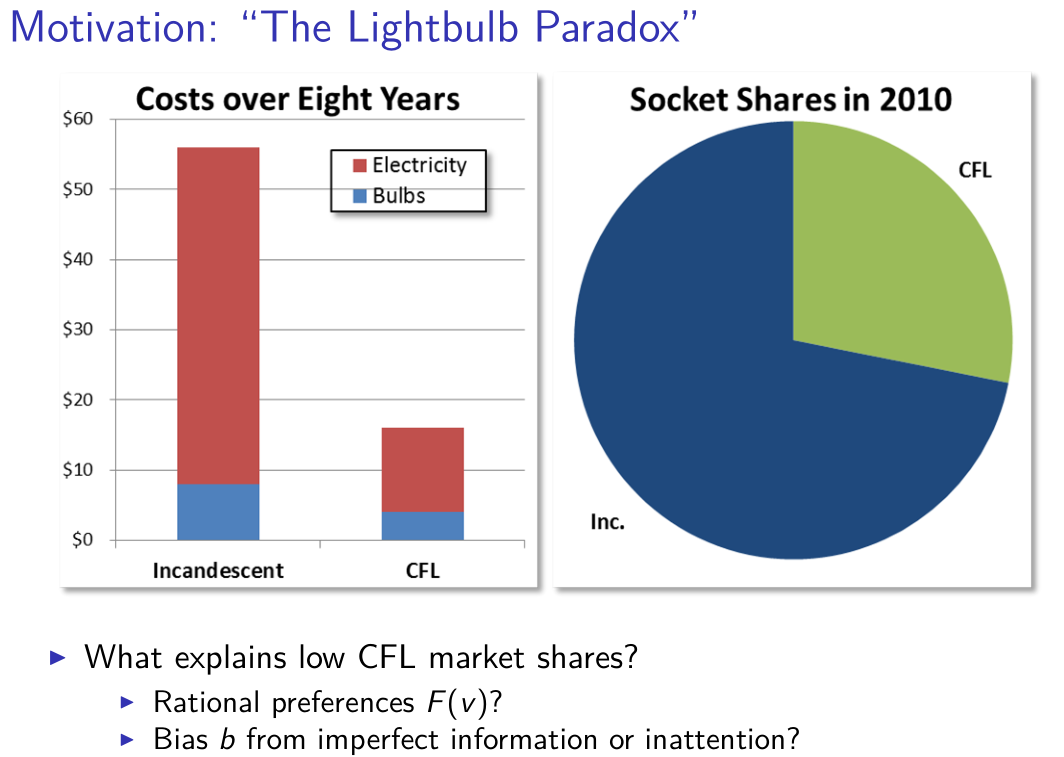### Can policymakers improve welfare in this market?

• develop a theoretical framework in presence of heterogenous bias and tastes

• implement two experiments informing consumers about CFLs

• evaluate welfare effects:

• optimal subsidy
• [ban on incandescents]

### Corrective taxation with damage heterogeneity

Pigou (1920):
$\tau = D'(e)$

If damages are heterogenous, first best achieved with polluter specific tax

$\tau_i = D_i'(e_i)$

Diamond (BJE 1973): If damages are heterogeneous, but you can only set one tax rate, it should be equal to the average damages at the margin when the tax is implemented.

$\tau_h = E_i[ D'_i(e_i(\tau_h))]$

### AT show this also applies to correcting biases

Setup

• unit demand: $j \in {E,I}$
• no outside option
• utility: $u_j = v_j + z - P_j$
• where $z$ is the consumer’s budget
• choose $E$ if $v - b > p$
• $v = v_E - v_I$
• $b$ is bias

### Demand with and without bias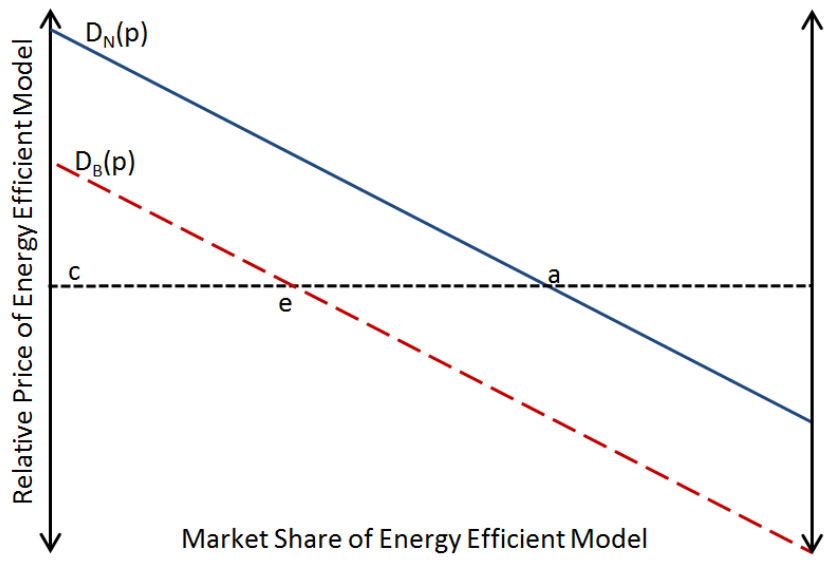### Welfare effect of subsidy

$W(s) = Z(s) + v_I - p_I + \int_{v-b \ge p}(v - p) dF dG$
• perfect competition: $p = c - s$

Proposition 1:

$W'(s) = (s - B(p)) D'_B(p)$
 where $B = E(b v - b = p)$ is the average marginal bias – ie the average bias for consumers on the margin the (subsidized) price

Optimal subsidy: $s^* = B(c - s^*)$

### Average Marginal Bias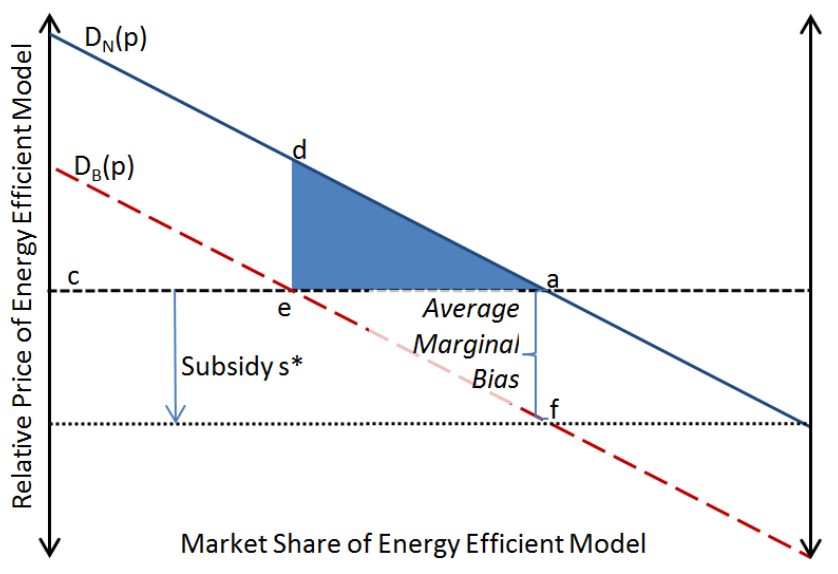### AMB is the key object of policy interest

Need not be constant

• Average marginal bias $\ne$ average bias

How can we estimate the average marginal bias?

Option 1:

• use within consumer variation in informedness

Option 2:

• estimate demand elasticity wrt tax and information
• calibrate difference

### TESS experiment

• Artefactual field experiment

### Bias is correlated with observables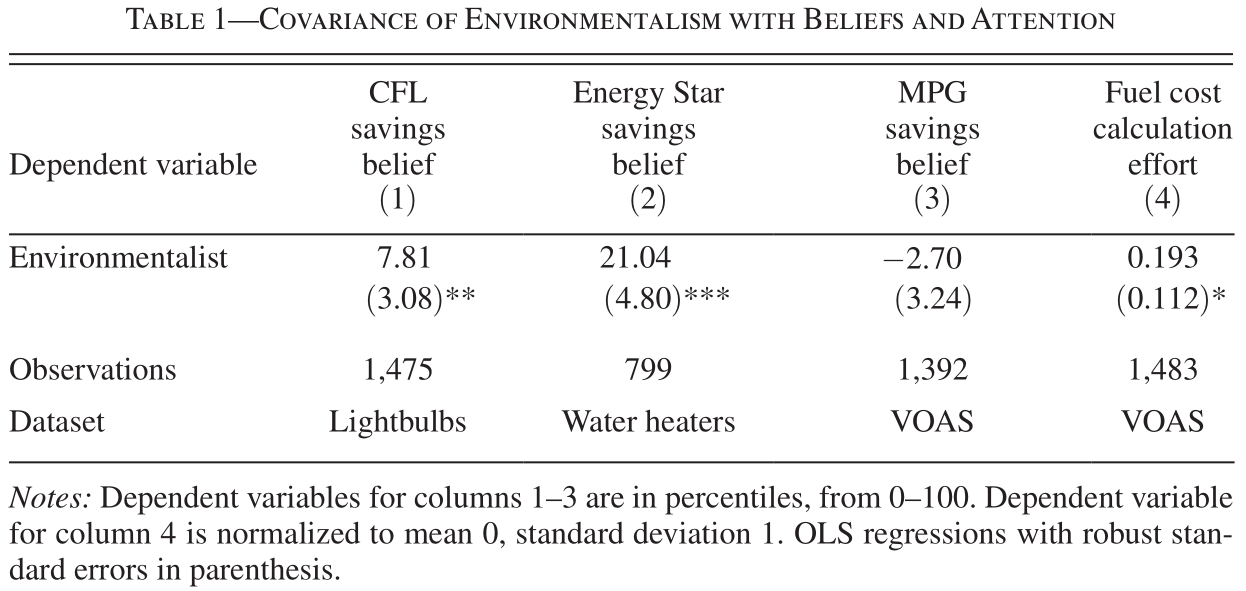### As is policy takeup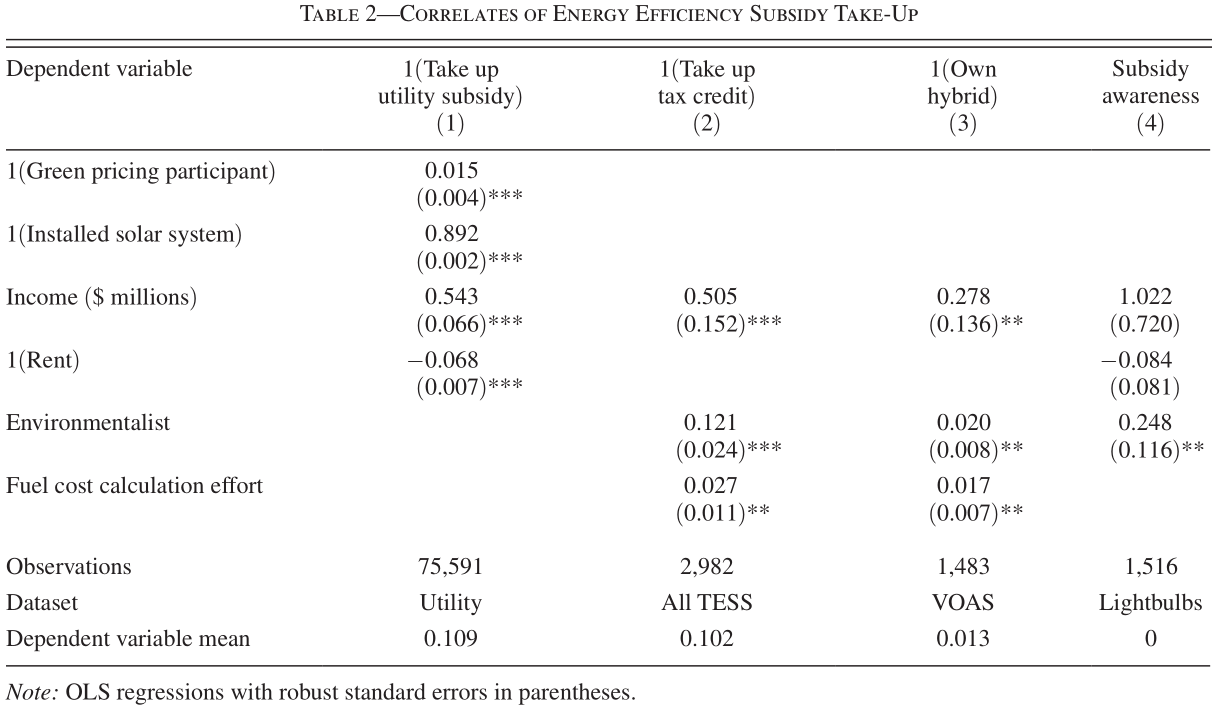### Where does this leave us?

• Optimal subsidy depends on average marginal bias

• Not sufficient to know bias and responsiveness separately: need to show biased consumers are actually the onces affected by the policy.

## Allcott and Sweeney

(MS 2017)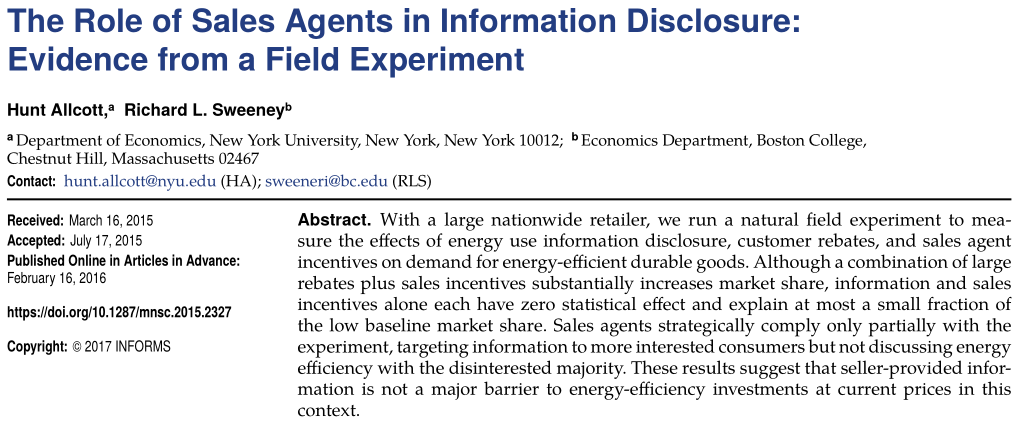### What we did

• partner with major appliance retailer
• field experiment at call center
• customer information and rebates
• sales agent incentives
• audit phone calls to check compliance
• survey consumers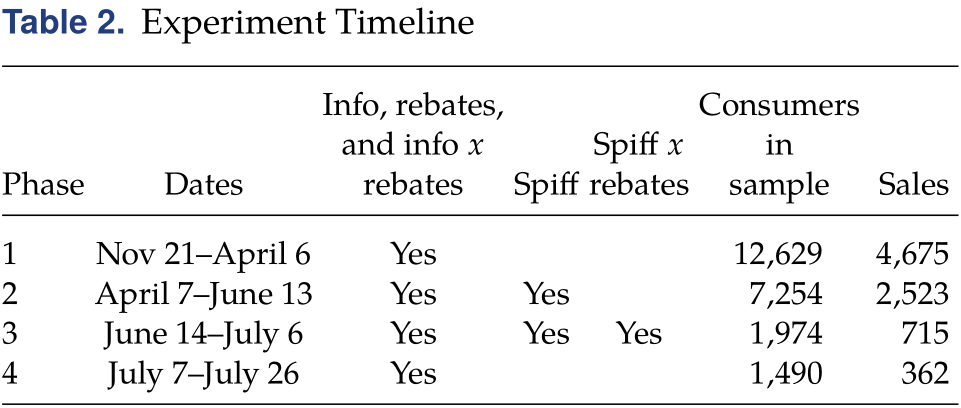### Some thoughts on running a field experiment

• getting institutional buy in was tough
• in end, partner lost interest
• compliance a big issue
• has implications for feasible policy too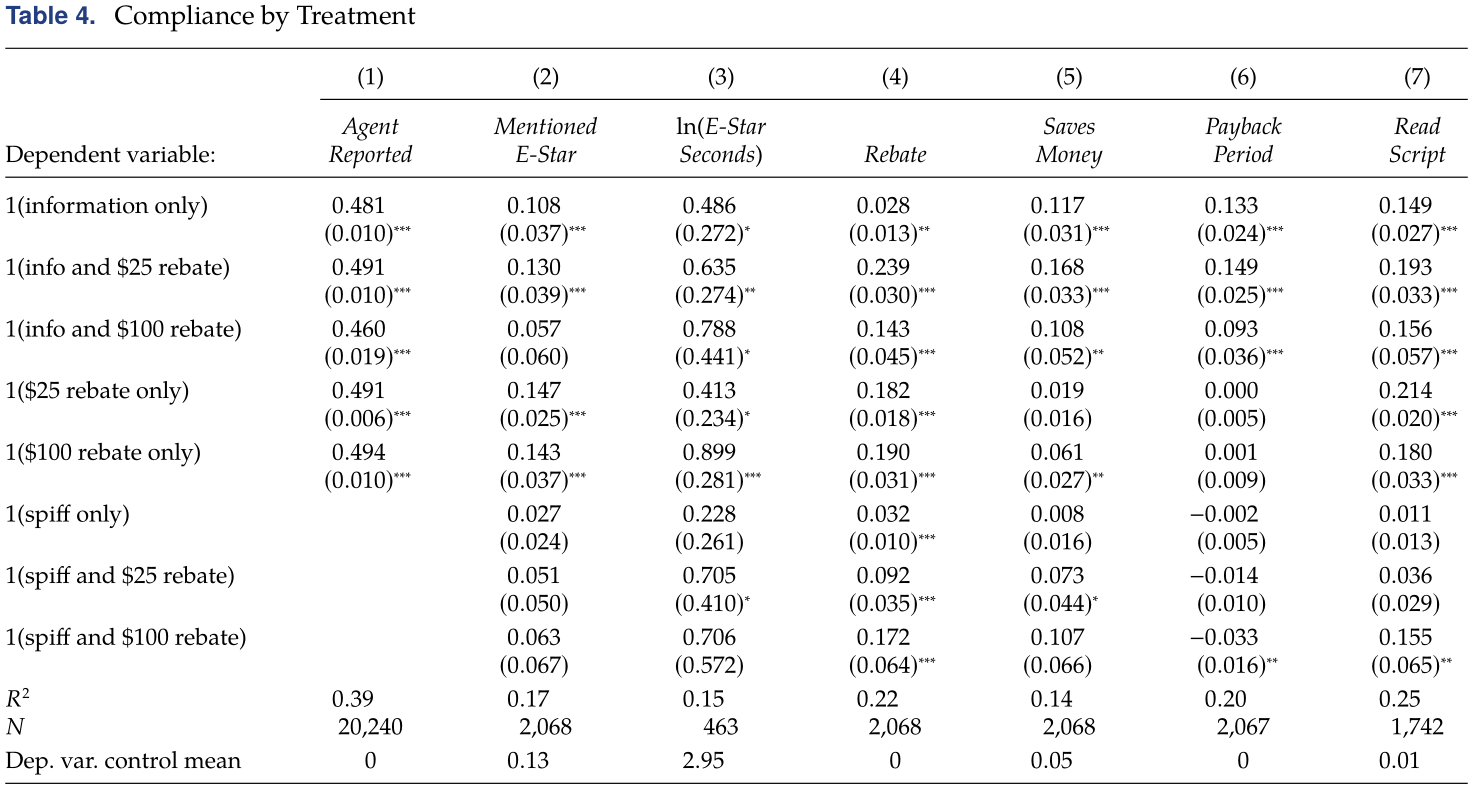### Some agents much better than others### Survey reveals widespread confusion, but small bias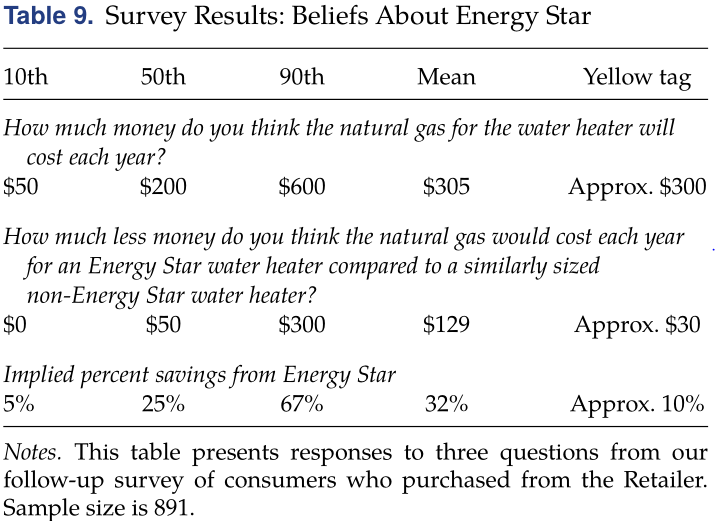### Main Results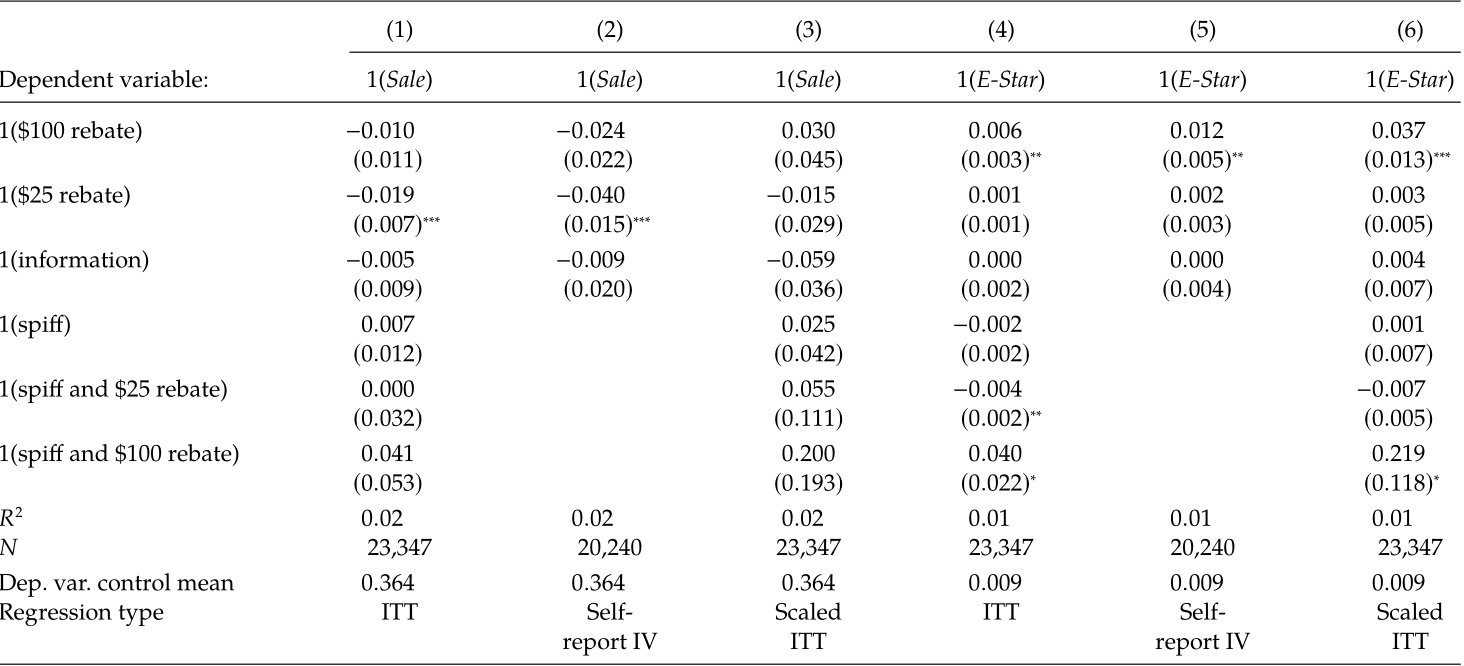### Agents Target Scripts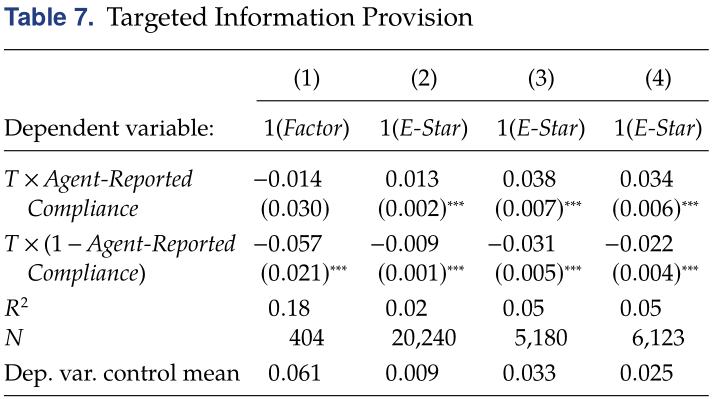### What role do firms play in this?

• Allcott & Sweeney: Sales agents can target. Can we leverage this somehow?

• Houde (2014): firms bunch product characteristics around subsidy / label cutoffs

• Houde (2018): labeling may further reduce average purchased quality

### Endogenous Attention?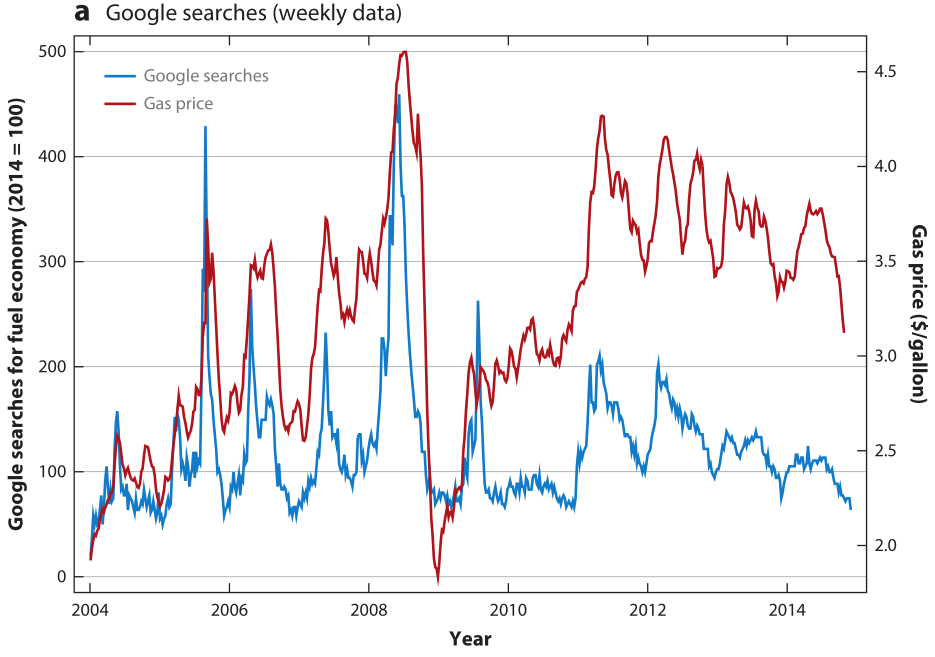### Endogenous Attention?